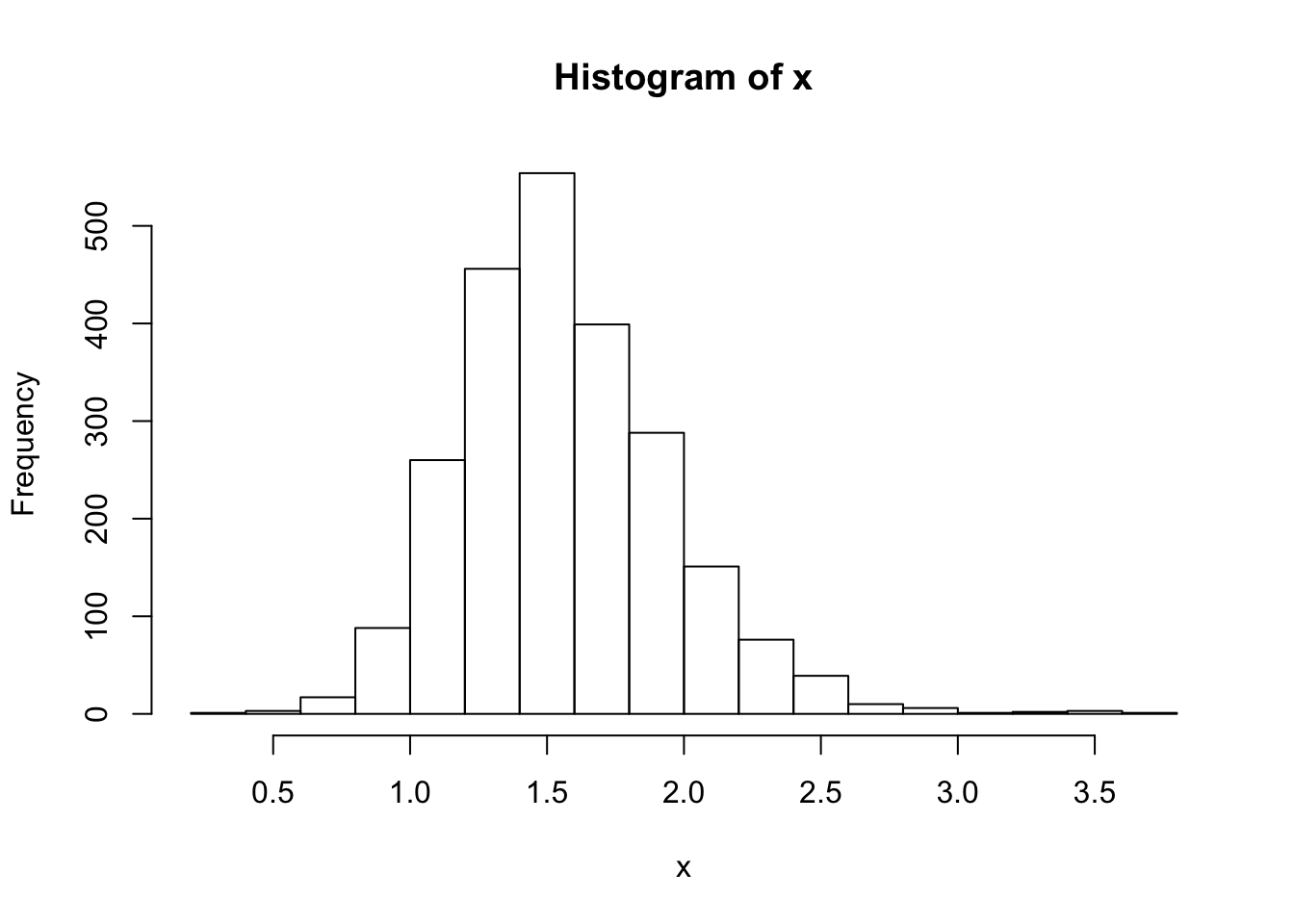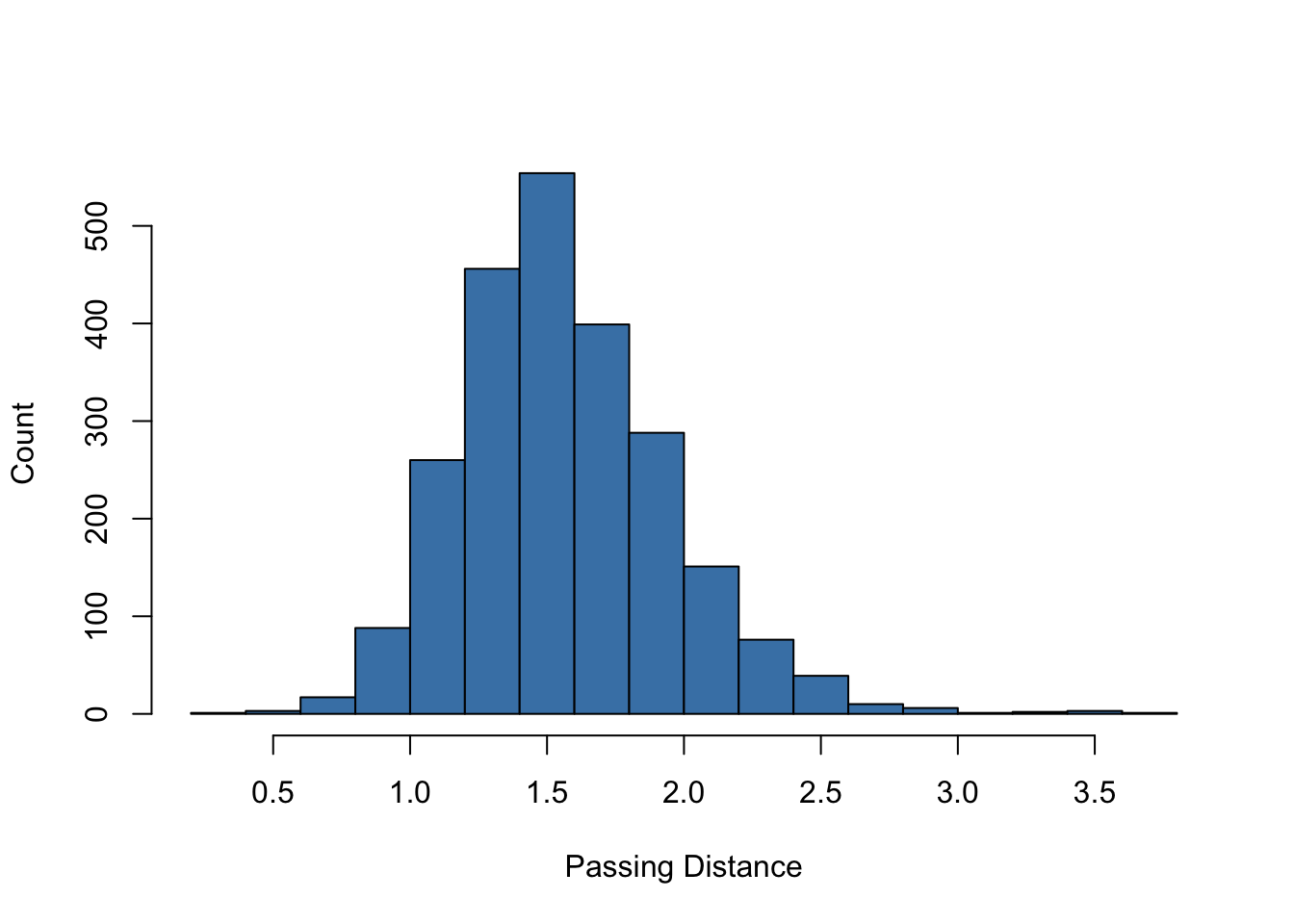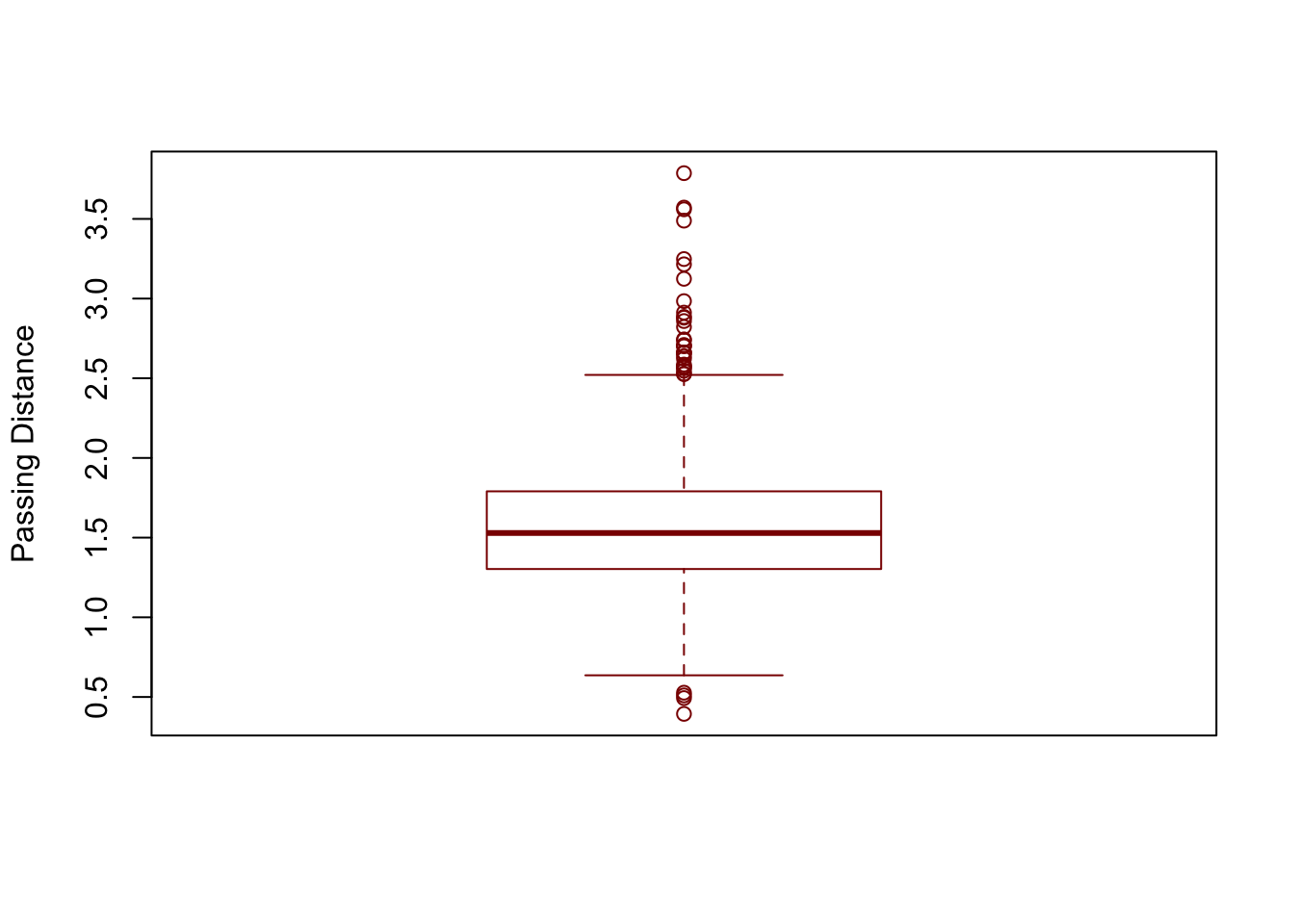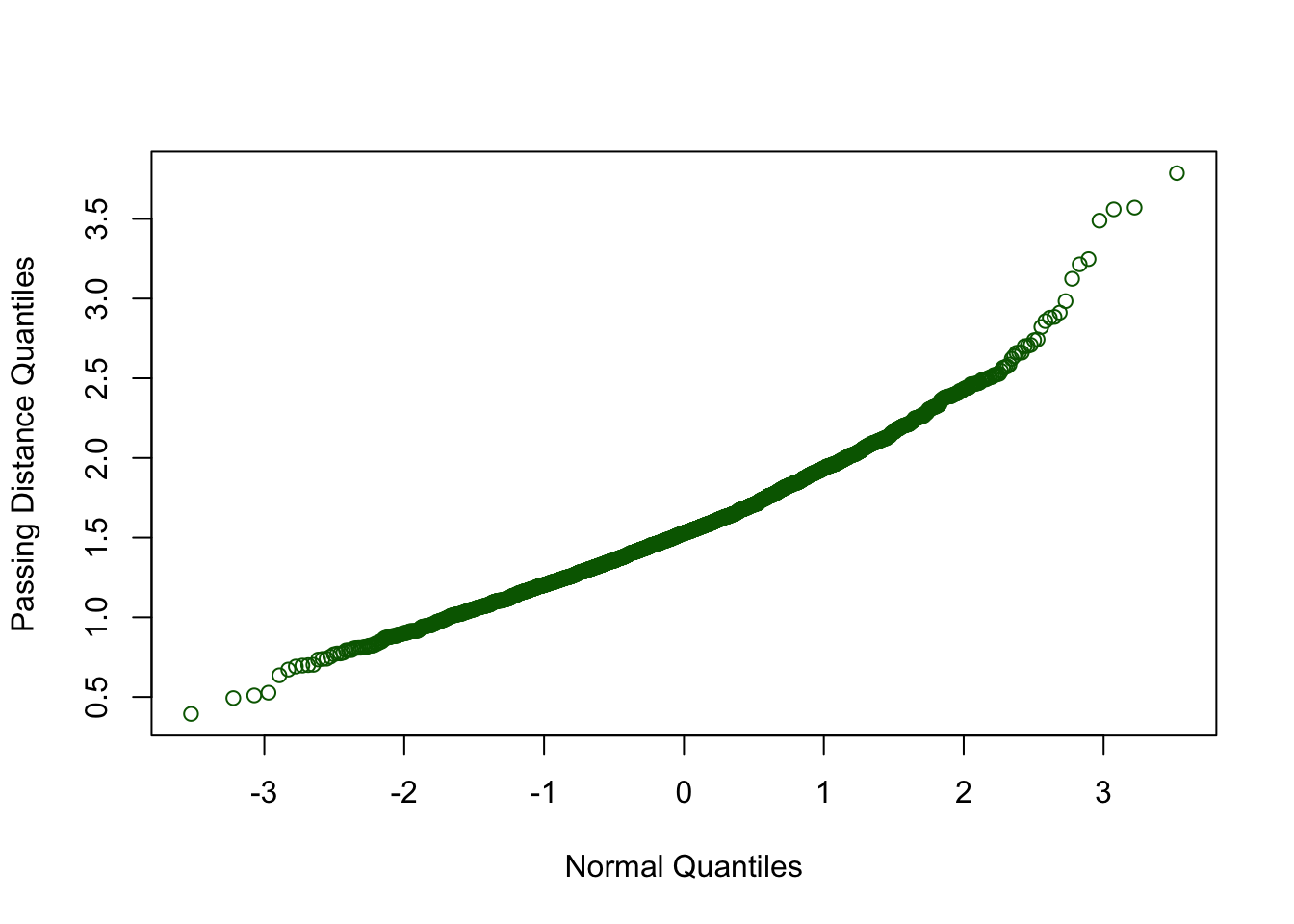## Preparing Data

Believe it or not, reading in data is often the hardest part of working with R. If you collect and store your data in Excel or Google Docs, you will need to carefully format your spreadsheet. It should obey the following rules:

1. The spreadsheet should contain a single sheet.

2. Row 1 should contain variable names in consecutive cells, starting with Cell A1. For convenience, the names should be comprised of lowercase words and contain no symbols or punctuation.

3. Subsequent rows (starting with Row 2) should contain your observations (data).

4. If the value of a variable is missing for a particular observation, the coressponding cell in the spreadsheet should be empty.

5. All other cells in the spreadsheet should be empty.

This sounds straightforward, but many spreadsheets that you find “in the wild” do not obey these rules. You will have to reformat these spreadsheets, usually by deleting empty rows and columns and by deleting notes and other annotations.

Even if your spreadsheet is formatted as above, R cannot open Excel files. To save your data to an R-compatible file format, exportyour data as a “Column-Separated Value” (CSV) file. You can do this from the File menu in Excel.

If you have a property-formatted CSV file, you can read it into R using the `read.csv` function. There are two ways to specify the file. To use your system’s file chooser, run the command.

``data <- read.csv(file.choose())``

Alternatively, if you know the name of the file, you can pass it directly to the `read.csv` function. Note that if you pass the file name directly, you must either specify the full path to the file, or you must set the “working directory” to be the directory thatcontains the file. To set the working directory, either use the `setwd` function or run the Set Working Directory command from RStudio’s Session menu.

Suppose that I want to open a file named “bikedata.csv”, which is stored in the “~/Datasets” directory on my system. I first set the working directory to “~/Datasets” by Choosing Session > Set Working Directory > Choose Directory …. This will execute the command

``setwd("~/Datasets")``

(In fact, if I do not want to use the menu system, then I can just type this command directly to achieve the same effect.) Once the working directory is set, I can read in the data to a variable named `data` by executing the command

``bikedata <- read.csv("bikedata.csv")``

One nice feature of the `read.csv` function is that it correctly web addresses. For example to read the file “bikedata.csv” from the course website, you can run the command

``bikedata <- read.csv("http://ptrckprry.com/course/forecasting/data/bikedata.csv")``

This downloads the file and reads the data into the `bikedata` variable.

# Basic Types and Operations

## Variables

In R, we use the term “variable” to refer to a name-value pair. You should not confuse this concept with the types of variables you have seen in your math classes (they are similar in some ways, but different in others).

In the last section, when we ran the command `data <- read.csv(file.choose())` we created a variable with name `data` and value equal to the contents of the chosen file.

To create a variable or to assign a new value to an existing variable, use the assignment command (`<-`), which is meant to look like an arrow pointing from the value to the variable name. For example, the command

``a <- 2.7``

means “assign the value `2.7` to the variable named `a`”. Another way to read this is “variable `a` gets the value `2.7`”.

When you have a variable, you can use the name in place of the value:

``a + 10``
``##  12.7``
``5 * a``
``##  13.5``

You can see the value of a variable, by typing its name and pressing enter:

``a``
``##  2.7``

An alternative way to read in a file to the variable named `data` is to run the following sequence of commands:

``````filename <- file.choose()

The first command asks the user to choose a file, and stores the resulting name in the `filename` variable. This variable contains the name of the file, but not the actual contents. The second command takes the name of the file, opens it, reads the contents into memory, and stores the result in the `data` variable.

## Functions

Besides variables, the other main concept you need to learn in R is that of a function. You are probably familiar with the concept of a function from your mathematics courses, and a function in R is very similar: a function is something that takes zero or more values, then performs a sequence of actions and returns a result.

We have already seen three functions: `file.choose`, `read.csv`, and `setwd`. We call a function by putting a pair of parentheses `()` after the function name. Many functions, including `read.csv` and `setwd` require one or more values as input. We refer to these values as arguments, and we specify them by putting the values inside the parentheses. When we do so, we say that we are passing the value of the argument to the function.

Sometimes a function will have optional arguments. These are arguments that, if left unspecified, will be given reasonable default values. For example, by default, the `file.choose` function forces the user to choose an existing file. To allow the user to choose a name for new file, pass the argument `new=TRUE` to file `file.choose` function:

``file.choose(new=TRUE)``

Before, we did not specify the `new` argument, and it defaulted to the value `FALSE`.

## Data Frames

The `read.csv` command opens the file and reads the data into a type of object called a ``dataframe’’. Conceptually, a data frame is just like a spreadsheet: it has columns, corresponding to variables, and rows, corresponding to observations. Each row and column has a name. Usually, the row names are the character strings “1”, “2”, etc., but this is not always the case.

To see the first 6 rows in the `bikedata` data frame, run the command

``head(bikedata)``
``````##   vehicle colour passing.distance street helmet kerb            datetime
## 1     Car   Blue            2.114  Urban      N  0.5 2006-05-11 16:30:00
## 2     HGV    Red            0.998  Urban      N  0.5 2006-05-11 16:30:00
## 3     LGV   Blue            1.817  Urban      N  0.5 2006-05-11 16:30:00
## 4     Car   <NA>            1.640  Urban      N  0.5 2006-05-11 16:30:00
## 5     Bus  Other            1.544  Urban      N  0.5 2006-05-11 16:30:00
## 6     Car   Grey            1.509  Urban      N  0.5 2006-05-11 16:30:00
##   bikelane      city
## 1        N Salisbury
## 2        N Salisbury
## 3        N Salisbury
## 4        N Salisbury
## 5        N Salisbury
## 6        N Salisbury``````

We can see that there are nine columns, named `vehicle`, `colour`, `passing.distance`, `street`, `helmet`, `kerb`, `datetime`, `bikelane`, and `city`.

To see a summary of the entire data frame, use the `summary` function:

``summary(bikedata)``
``````##  vehicle         colour    passing.distance         street     helmet
##  Bus :  46   Blue   :636   Min.   :0.394    Main       :1637   N:1206
##  Car :1708   Grey   :531   1st Qu.:1.303    OneWay1    :   9   Y:1149
##  HGV :  82   Red    :378   Median :1.529    OneWay2    :  13
##  LGV : 293   White  :333   Mean   :1.564    Residential:  39
##  PTW :  34   Black  :262   3rd Qu.:1.790    Rural      :   2
##  SUV : 143   (Other):201   Max.   :3.787    Urban      : 655
##  Taxi:  49   NA's   : 14
##       kerb                       datetime    bikelane        city
##  Min.   :0.2500   2006-05-20 16:21:00:  93   N:2305   Bristol  : 450
##  1st Qu.:0.2500   2006-05-20 15:48:00:  75   Y:  50   Salisbury:1905
##  Median :0.5000   2006-05-31 09:04:00:  70
##  Mean   :0.6702   2006-05-20 15:34:00:  65
##  3rd Qu.:1.0000   2006-05-27 10:01:00:  64
##  Max.   :1.2500   2006-05-27 09:25:00:  63
##                   (Other)            :1925``````

## Extracting Columns

Let’s say we want to investigate the `passing.distance` variable. To do this, we must first extract that column from the `bikedata` dataframe. There are three ways to do this:

``````x <- bikedata\$passing.distance
x <- bikedata[["passing.distance"]]
x <- bikedata[,"passing.distance"]``````

All three commands are equivalent ways to extract the `passing.distance` column and store it in a variable named `x`. The `\$` form is the most common, but you will sometimes see the other two forms, as well.

## Vectors

Data frame columns are stored in a data type called a “vector”. Conceptually, a vector is a one-dimensional array of values, indexed by integers starting at `1`. Most functions in R operate on vectors.

You can access individual values by using double square-brackets. For example, to see the first element of the vector, type the command

``x[]``
``##  2.114``

To see the fifth value, type the command

``x[]``
``##  1.544``

To see how many elements are contained in the vector, use the `length` function:

``length(x)``
``##  2355``

To see the last element, type

``x[[length(x)]]``
``##  1.031``

To extract a subvector, use single square brackets. For example, the subvector consisting of the first 25 elements is

``x[1:25]``
``````##   2.114 0.998 1.817 1.640 1.544 1.509 1.290 1.512 1.049 1.932 1.145
##  1.410 1.428 1.494 1.570 2.103 0.896 1.160 1.290 1.963 2.436 2.304
##  1.482 1.492 1.432``````

Here, `1:25` is shorthand for “integers 1 to 25”. Since not all 25 values fit onto a single line, R wraps the values. At the start of each line, R prints the index of the first value on the line in square brackets. Looking at the output above, we can see that `1.410` is the 12th element and `1.492` is the 24th element of the result.

You may have asked yourself earlier why the output of `x[]` and other similar commands was prefixed by ``. The reason for this is that R doesn’t have the concept of a “single value” or “scalar”. The only way to represent the value of `x[]` is as a length-one vector. The output

``` 2.114
```

denotes a vector with a single value (`2.114`), stored at index `1`.

Since there is no concept of a “scalar” in R, the command `x[]` is equivalent to `x[1:1]`, which is also the same as `x`. In other programming languages, `x[]`, “the first element of `x`”, and `x`, “the subvector of `x` starting and ending at index `1`” would be different; in R, these are identical. Because of this, most people use single brackets instead of double brackets when indexing vectors, writing `x` and `x` instead of `x[]` and `x[]]`.

# Plots and Descriptive Statistics

## Descriptive Statistics for Numeric Variables

There are a variety of functions for computing descriptive statistics for the values stored in a vector.

• Sum of values:

``sum(x)``
``##  3683.013``
• Measures of central tendency (sample mean and median):

``mean(x)``
``##  1.563912``
``median(x)``
``##  1.529``
• Measures of variability (sample standard deviation and sample variance):

``sd(x)``
``##  0.3834545``
``var(x)``
``##  0.1470373``
• Extreme values (minimum and maximum):

``min(x)``
``##  0.394``
``max(x)``
``##  3.787``
• Quantiles:

``quantile(x, .25) # first quartile``
``````##   25%
## 1.303``````
``quantile(x, .75) # third quartile``
``````##    75%
## 1.7905``````
``quantile(x, .99) # 99th percentile``
``````##     99%
## 2.58006``````

## Plots for Numeric Variables

We can use the `hist` command to make a histogram of the values stored in a vector:

``hist(x)``By default, the output looks fine when printed in black and white, but it isn’t very pretty. We can specify the bin color, change the axis labels, and omit the main title by passing additional arguments to this `hist` function

``````hist(x, col="steelblue", xlab="Passing Distance", ylab="Count",
main="")``````Use the `boxplot` and `qqnorm` commands to make boxplots and normal probability plots, as in the following examples:

``boxplot(x, border="darkred", ylab="Passing Distance")````````qqnorm(x, col="darkgreen", xlab="Normal Quantiles",
ylab="Passing Distance Quantiles",
main="")``````## Scatterplots

We can make a scatter plot using the `plot` command. For example, to plot passing distance versus distance to the kerb, run the command

``````plot(bikedata\$kerb, bikedata\$passing.distance,
xlab="Distance to Kerb",
ylab="Passing Distance")``````